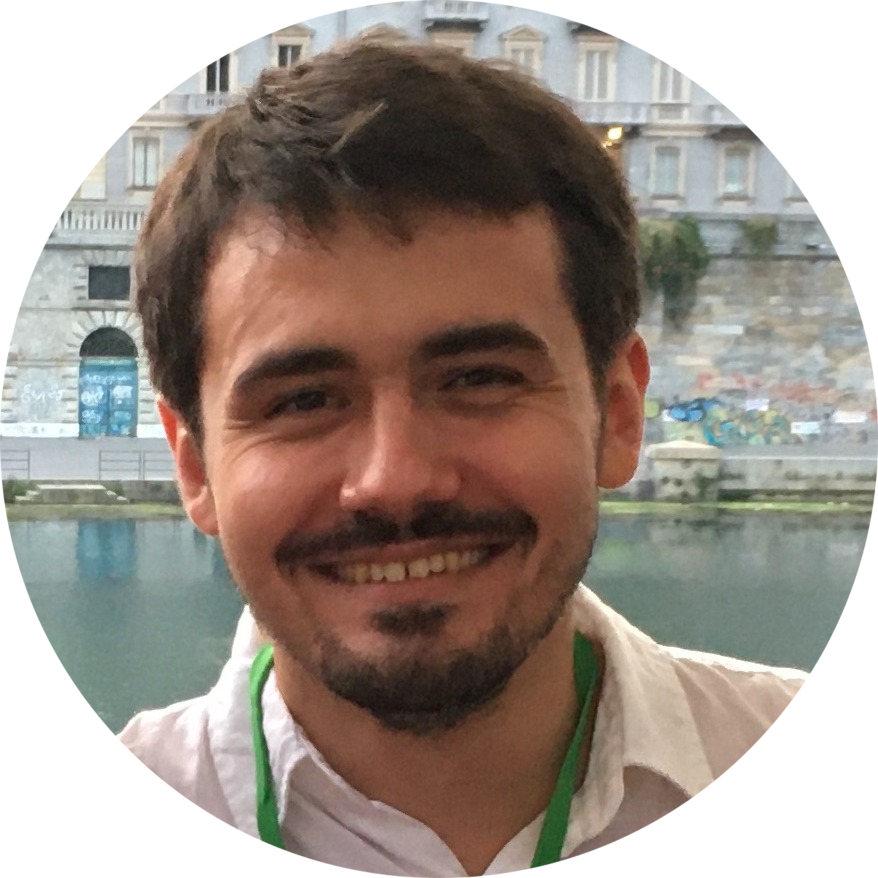INSTITUTE FOR THEORETICAL AND MATHEMATICAL PHYSICS

Lomonosov Moscow State UniversityDmitri Bykov

PhD

#### Research interests

My scientific interests are related to the application of geometric methods in theoretical/mathematical physics. Results are in the theory of flag manifold sigma models, integrable sigma models, as well as in the construction of Ricci-flat metrics on non-compact Kähler manifolds and in the study of mathematical aspects of Nekrasov-Shatashvili theory. In particular, I showed that certain spin chains with SU(N) symmetry are described, in a continuum limit, by flag manifold sigma models, which later on led to a formulation of the so-called generalized Haldane conjectures for such systems by I.Affleck et.al. In the theory of integrable models I formulated a conjecture about the integrability of certain models with non-symmetric target spaces (such as flag manifolds). It was subsequently found that such models naturally fit in a general framework developed by C.Costello and M.Yamazaki and that, in fact, there is a wider class of integrable models with quiver (super)variety phase spaces, which from a physics perspective are equivalent to generalized Gross-Neveu models with Bose- and Fermi- fields.

Publications
Teaching

#### Selected publications:

1. I.Affleck, D.Bykov, K.Wamer "Flag manifold sigma models: spin chains and integrable theories", to appear in Phys.Rept., arXiv:2101.11638.
2. D. Bykov, "The CP^{n−1}-model with fermions: a new look", to appear in Adv.Theor.Math.Phys., arXiv:2009.04608.
3. D. Bykov, "Quantum flag manifold sigma-models and Hermitian Ricci flow", to appear in Comm.Math.Phys., arXiv:2006.14124.
4. I. Achmed-Zade, D. Bykov, "Ricci-flat metrics on vector bundles over flag manifolds", Comm. Math. Phys., 376:3 (2020), 2309–2328 , arXiv:1905.00412.
5. D. Bykov, P. Zinn-Justin, "Higher spin sl2 R-matrix from equivariant (co)homology", Lett. Math. Phys., 110 (2020), 2435–2470 , arXiv:1904.11107.
6. Dmitri Bykov, "Complex structures and zero-curvature equations for sigma models", Phys. Lett. B, 760 (2016), 341–344, arXiv:1605.01093.
7. D. Bykov, "Integrable properties of sigma models with non-symmetric target spaces", Nuclear Phys. B, 894 (2015), 254–267 , arXiv:1412.3746.
8. D. Bykov, K. Zarembo, "Ladders for Wilson loops beyond leading order", J. High Energy Phys., 2012, no. 9, 057 , 14 pp., arXiv:1206.7117.
9. D. Bykov, "The geometry of antiferromagnetic spin chains", Comm. Math. Phys., 322:3 (2013), 807–834 , arXiv:1206.2777.
10. D. Bykov, "Haldane limits via Lagrangian embeddings", Nuclear Phys. B, 855:1 (2012), 100–127 , arXiv:1104.1419.
11. D. Bykov, "The worldsheet low-energy limit of the AdS4×CP3 superstring", Nuclear Phys. B, 838:1-2 (2010), 47–74, arXiv:1003.2199.
12. L. F. Alday, G. Arutyunov, D. Bykov, "Semiclassical quantization of spinning strings in AdS_4×CP^3", J. High Energy Phys., 2008, no. 11, 089 , 21 pp., arXiv:0807.4400.

#### Publications affiliated with ITMP:

1. Bykov, D. (2022). "Integrable sigma models on Riemann surfaces". arXiv: 2202.12805 [hep-th].

All publications

Dmitri Bykov at Steklov Institute of Mathematics RAS

physics and mathematics